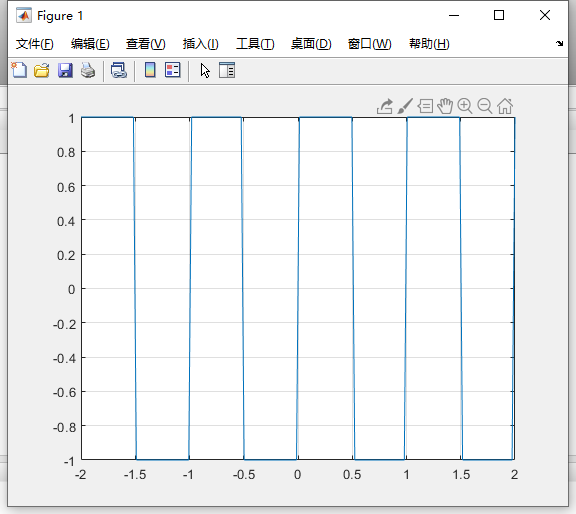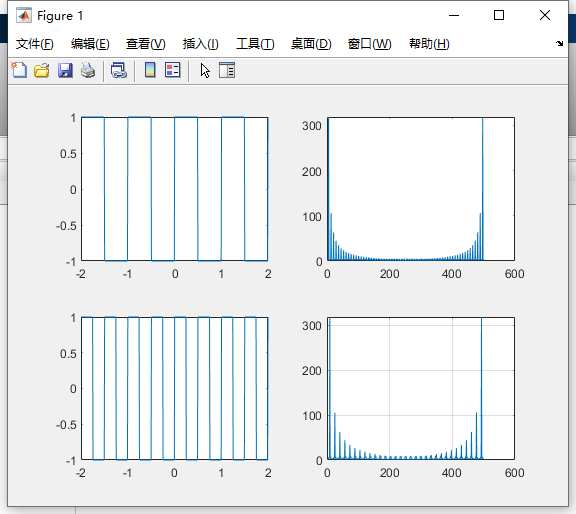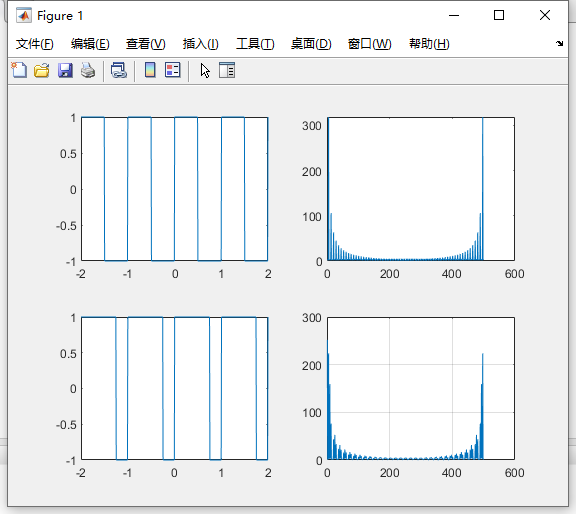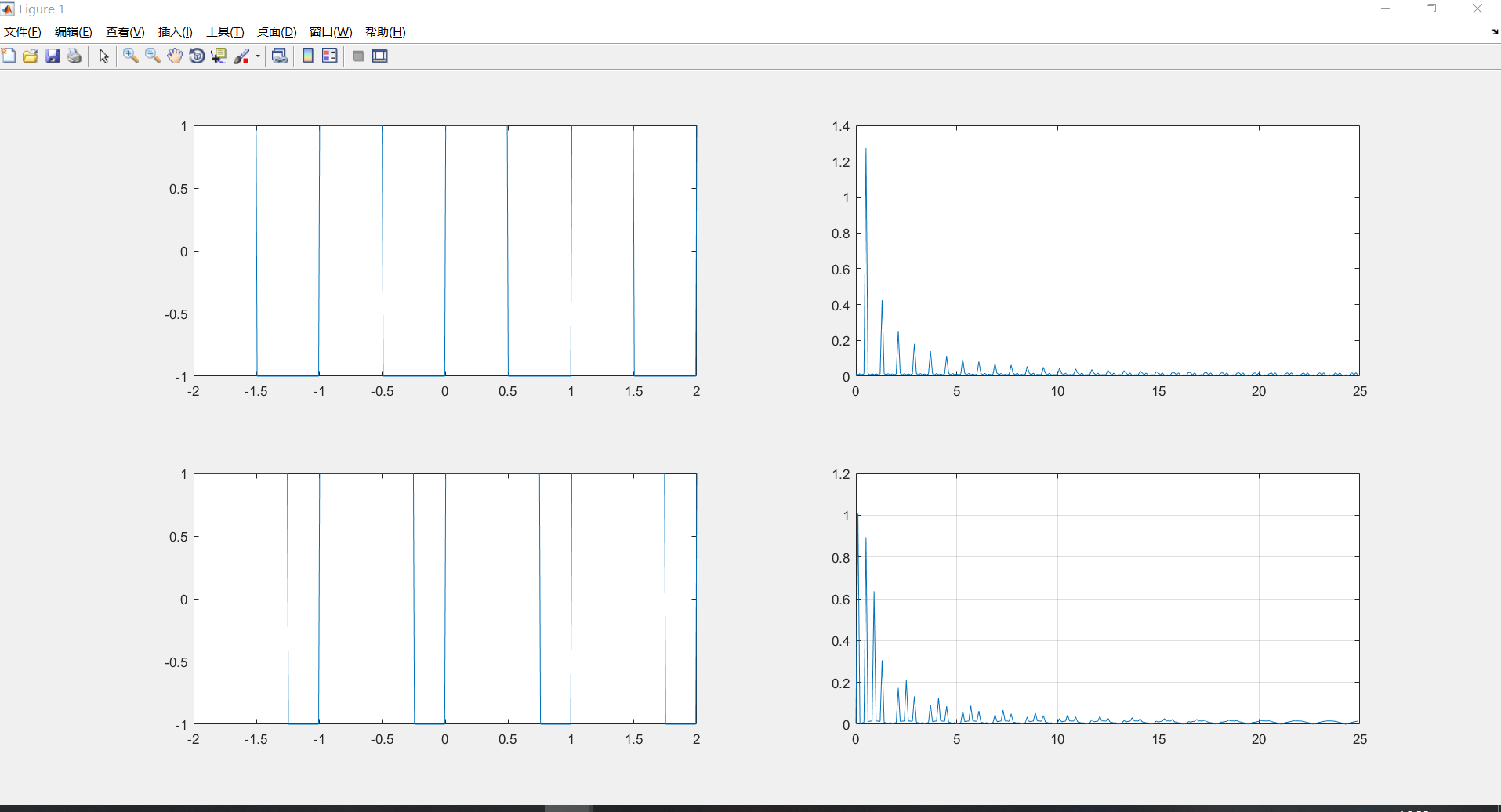• 主要介绍了python 周期方波信号频谱图，小编觉得挺不错的，现在分享给大家，也给大家做个参考。一起跟随小编过来看看吧
• 文档是PDF格式，写了周期方波信号频谱图和傅里叶变换推导过程方波频谱图
• 基于MATLAB仿真的连续信号频谱分析.doc.doc实验十：基于MATLAB仿真的连续信号频谱分析一、实验目的1、学会运用MATLAB求连续时间信号的傅里叶变换；2、学会运用MATLAB求连续时间信号的频谱图。二、实验原理1、周期...

基于MATLAB仿真的连续信号频谱分析.doc.doc

实验十：基于MATLAB仿真的连续信号频谱分析

一、实验目的

1、学会运用MATLAB求连续时间信号的傅里叶变换；

2、学会运用MATLAB求连续时间信号的频谱图。

二、实验原理

1、周期信号的分解

根据傅里叶级数的原理，任何周期信号都可以表示为三角级数的组合称为f(t)的傅

合成波形所包含的谐波分量越多，除间断点附近外，它越接近于原波形，在间断点附近，即使合成的波形所含谐波次数足够多，也仍存在约9%的偏【示例1】用正弦信号的叠加近似合成一个频率为50Hz，幅度为3的MATLAB程序如下：

clear all;

fs=5000;

t=0:1/fs:0.1;

f0=50;sum=0;

subplot(211)

for n=1:2:9

plot(t,4/pi*1/n*sin(2*pi*n*f0*t),'k');

hold on;

end

title('信号叠加前');

subplot(212)

for n=1:2:9;

sum=sum+4/pi*1/n*sin(2*pi*n*f0*t);

end

plot(t,sum,'k')

10.1所示。

2、非周期信号的频谱分析

信号的傅里叶变换定义为

(.1)

傅里叶反变换定义为

(.2)

信号的傅里叶变换主要包括MATLAB符号运算和MATLAB数值分析两种方法。

(1) MATLAB符号运算求解法

MATLAB符号数学工具箱提供了直接求解傅里叶变换和傅里叶反变换的函数fourier( )及ifourier( )。傅里叶变换的语句格式分为三种。

F=fourier(f )；表示符号函数f的Fourier变换，默认返回是关于的函数。

F=fourier(f ,v)；表示返回函数F是关于符号对象v的函数，而不是默认的，即。

F=fourier(f ,u,v); 是对关于u的函数f进行变换，返回函数F是关于v的函数，即。

类似的，傅里叶反变换的语句格式也分为三种。

要注意的是，函数fourier( )及ifoureir( )都是接收由sym函数所定义的符号变量或者符号表达式。【2】用MATLAB符号运算求解法求单边指数信号的傅里叶变换。

解：MATLAB源程序为

>> ft=sym('exp(-2*t)*Heaviside(t)');

>> Fw=fourier(ft)

运行结果为

Fw =

1/(2+i*w)

(2) 连续时间信号的频谱图

信号f(t)的傅里叶变换表达了信号在处的频谱密度分布情况，这就是信号的傅里叶变换的物理意义。一般是复函数，可以表示为。我们把与曲线分别称为非周期信号的幅度频谱和相位频谱。

【3】用MATLAB命令绘制单边指数信号幅度谱和相位谱。

解：MATLAB源程序为

ft=sym('exp(-2*t)*Heaviside(t)');

Fw=fourier(ft);

subplot(211)

ezplot(abs(Fw),[-2*pi,2*pi]),grid on

title('幅度谱')

phase=atan(imag(Fw)/real(Fw));

subplot(212)

ezplot(phase,[-2*pi,2*pi]),grid on

title('相位谱')

程序运行结果如图.2所示。

(3)MATLAB数值计算求解法

Fourier( )和ifourier( )函数的一个局限性是，如果返回函数中有诸如狄拉克函数等项，则用ezplot( )函数无法作图。对某些信号求变换时，其返回函数可能包含一些不能直接用符号表达的式子，甚至可能出现提示“未被定义的函数或变量”，因而也不能对其返回函数作图。此外，在很多实际情况中，尽管信号是连续的，但经过抽样所获得的信号则是离散的数值量，因此无法表示成符号表达式，此时不能应用Fourier( )函数对进行处理，而只能用数值计算法来近似求解。下面介绍傅里叶变换的数值计算法。

从傅里叶变换定义出发，有

(3)

当足够小时，上式的近似情况可以满足实际需要。对于时限信号，或者在所研究的时间范围内衰减到足够小，上式可研究有限n的取值。假设是因果信号，则有

(4)

对(4)式的角频率进行离散化，假设离散化后得到N个样值，即

， (5)

因此有

采用行向量，用矩阵表示为

(6)

式(6)提供了用MATLAB实

展开全文• 18. Scipy Tutorial- 方波傅里叶分解与合成周期性矩形波(方波)信号：在scipy中用signal包里的square函数来表示。signal里支持的内置其他波形函数可以到其官网查找。当然构造一个方波也可用numpy的zeros和ones来构建...

18. Scipy Tutorial- 方波傅里叶分解与合成

周期性矩形波(方波)信号：在scipy中用signal包里的square函数来表示。signal里支持的内置其他波形函数可以到其官网查找。当然构造一个方波也可用numpy的zeros和ones来构建。

#coding:utf-8

import numpy as np

import matplotlib.pyplot as plt

x = np.zeros(500)

x[100:150] = 1

plt.plot(x)

plt.ylim(-0.3, 1.3)

plt.show()根据傅立叶分析，任何信号都可以分解成一系列不同频率的正弦信号，方波中包含了非常丰富的频谱成分。下面可以用实验来直观的分析方波中的频率成分即fft，看看不同频率的正弦信号是如何叠加成为方波的即ifft。

#coding:utf-8

import numpy as np

from scipy import fftpack,signal

import matplotlib.pyplot as plt

b = 30

f_s = 80

N = 8000

t = np.linspace(0, 10, N, endpoint=False)

sq = signal.square(2 * np.pi * 5 * t)

F = fftpack.fft(sq)

f = fftpack.fftfreq(N, 1.0/f_s)

F_filtered = F * (abs(f) < 5)

print "F_filtered", F_filtered

ift = fftpack.ifft(F_filtered)

fig, axes = plt.subplots(4,1)

axes.plot(t, sq)

axes.set_ylim(-2, 2)

axes.plot(t,ift.real, label="all")

axes.plot(t,ift.real, label="zoom")

axes.set_xlim(1, 2)

plt.show()

整个程序有三部分：

(1).利用scipy.signal包里的square函数生成方波。

sq = signal.square(2 * np.pi * 5 * t)

结果如下图子图1,最顶上的图。

(2).利用scipy.fftpack包里的fft对方波进行频谱分析，即分解得到若干个频率。

F = fftpack.fft(sq)

f = fftpack.fftfreq(N, 1.0/f_s)

可视化图为下图子图2,即第二个图。

(3). 利用scipy.fftpack包里的ifft快速傅里叶逆变化得到若干频率正弦波组成的时域信号。结果如下图子图3和4,子图4是对子图3的局部放大。展开全文python画方波
• I need to plot the frequency spectrum for a square wave using MATLAB. The wave is HIGH (5mV) between 0 and -2 and LOW (omv) between 0 and 2. I have already obtained the fourier seires for this functio...

I need to plot the frequency spectrum for a square wave using MATLAB. The wave is HIGH (5mV) between 0 and -2 and LOW (omv) between 0 and 2. I have already obtained the fourier seires for this function and i have the first ten components of the series.

(5/2) + ((10/pi)*sin((pi*t)/2)) + ((10/(3*pi))*sin((3*pi*t)/2)) + ((10/(5*pi))*sin((5*pi*t)/2)) + ((10/(7*pi))*sin((7*pi*t)/2))+ ((10/(9*pi))*sin((9*pi*t)/2))+ ((10/(11*pi))*sin((11*pi*t)/2))+ ((10/(13*pi))*sin((13*pi*t)/2))+ ((10/(15*pi))*sin((15*pi*t)/2))+ ((10/(17*pi))*sin((17*pi*t)/2))+ ((10/(19*pi))*sin((19*pi*t)/2))

How do I plot the frequency spectrum for this wave using MATLAB? I have tried using FFTs, but I really don't know how it works to plot the graph.

展开全文•python
• 实现频谱分析。能显示信号频谱图。主要是对正弦信号和三角进行一维FFT变换，得到频谱图
• matlab关于加均匀噪声、高斯噪声、粉红噪声的信号，分别画出其频谱图、对数谱、功率谱
• 方波生成主要是函数square的运用。可直接 help square看到相关的代码 t = linspace(-2*pi,2*pi,150);%150是从-2π到2π取150个点，可取其他数字，不写默认为100 x = square(2*t);%数字2可改变方波的周期。另外可...

方波生成主要是函数square的运用。可直接 help square看到相关的代码

t = linspace(-2*pi,2*pi,150);%150是从-2π到2π取150个点，可取其他数字，不写默认为100
x = square(2*t);%数字2可改变方波的周期。另外可square（2*t，75）改变方波占空比，使得方波正的部分的占空比为75%
plot(t/pi,x)
grid ont的区间内取点个数越大方波越正，默认为100的时候有点斜。

t = linspace(-2*pi,2*pi,500);
x = square(4*t);%周期相关
subplot(2,3,1)
plot(t/pi,x)
subplot(2,3,2)
plot(abs(fft(x)))
grid on

周期的影响：

改变周期做对比可发现，系数变大，周期变小，谱线间隔变大，频谱变稀疏脉宽的影响：

脉宽由信号的周期和占空比确定，其计算公式是脉宽W=T×P（T：周期，P：占空比）。固定周期，增加占空比到75如下图所示可看出脉宽增加的时候频谱的谱线间隔变小，频谱变稠密。此时可以明显的看到频谱的幅度也减小，不知道事实如此还是我代码有问题，没检索到相关内容佐证

------------------------------------------------按照大佬的思路，加入了幅值矫正和调整0频位置

t = linspace(-2*pi,2*pi,500);
x = square(2*t);
N = length(x);
Fs = 50;%设定采样频率50hz
f = (0:N-1)*Fs/N;%将时间横坐标转换为频率横坐标：f = (0:N-1)*Fs/N
f2 = (N/2:N-1)*Fs/N-Fs/2;
subplot(2,2,1)
plot(t/pi,x)
subplot(2,2,2)
y = abs(fftshift(fft(x)));
y2=2*y(N/2:N-1)/N;%幅值修正得到真实幅值
plot(f2,y2)
x = square(2*t,75);
y = abs(fftshift(fft(x)));
y2=2*y(N/2:N-1)/N;
subplot(2,2,3)
plot(t/pi,x)
subplot(2,2,4)
plot(f2,y2)
grid on
% plot((0:7)*0.0001,[0 0.5 1 1 1 1 0.5 0])参考资料：

square的MATLAB解释

MATLAB绘制频谱图代码

展开全文数字信号处理
• 满采样模拟f1=12.5M，f2=10.9375M，f3=12.3046785M三个方波信号，并通过“与”操作混合三信号。f1=100/8； .5Mf2=700/64； .9375Mf3=6300/512； .3046785Mff=787.5； %三个频率的公倍数作采样频率A=ff/f1； %若按照...
• 主要介绍了Python实现正弦信号的时域波形和频谱图,涉及Python数学运算与图形绘制相关操作技巧,需要的朋友可以参考下
• 基于MATLAB仿真的连续信号频谱分析.doc.doc实验十：基于MATLAB仿真的连续信号频谱分析一、实验目的1、学会运用MATLAB求连续时间信号的傅里叶变换；2、学会运用MATLAB求连续时间信号的频谱图。二、实验原理1、周期...
• 一、实验目的： 1、掌握傅立叶级数（FS），学会分析连续时间周期信号频谱分析及MATLAB实现； 2、掌握傅立叶变换（FT），了解傅立叶变换的性质以及MATLAB实现。 二、利用符号运算求傅里叶级数的系数 1、复习几个...
• 周期锯齿傅立叶级数（离散频谱）的MATLAB实现，绘制并观察周期锯齿脉冲信号频谱特性，可自改成其他周期函数
• 2上式可以看成周期离散信号x(n)的离散傅里叶级数展开。????(????)=∑????(????????)????????????????????????−1????=0上式是DFS的反变换，记作IDFS并且称X(kΩ)与x(n)构成一对离散傅里叶级数变换对。(以上两式中Ω=...
• 2．进行FFT变换，显示各自频谱图，其中采样率，频率、数据长度自选 3．做出上述三种信号的均方根图谱,功率图谱,以及对数均方根图谱 4．用IFFT傅立叶反变换恢复信号，并显示恢复的正弦信号时域波形图频谱分析
• 1-1求周期方波(见1-4)的傅里叶级数.doc1-1 求周期方波(见1-4)的傅里叶级数(复指数函数形式)，划出|cn|–ω和φn–ω，并与表1-1对比。解答：在一个周期的表达式为积分区间取(-T/2，T/2)所以复指数函数形式的...
• 1-1 求周期方波(见1-4)的傅里叶级数(复指数函数形式)，划出|cn|–ω...T0 1-4 周期方波信号波形解答：在一个周期的表达式为T0??A (??t?0)??2 x(t)??? A (0?t?T0)??2积分区间取(-T/2，T/2)T02T?02T0201cn?T0 =......